CELIA3D  1.0 Fluid-structure interaction using cut-cells
couplage.cpp File Reference

Definition of specific coupling functions. More...

#include "fluide.hpp"
#include "intersections.cpp"

## Functions

double volume_prisme (const Triangle_3 &T1, const Triangle_3 &T2)
Computation of the signed volume of a prism. More...

double volume_tetra (const Tetrahedron &Tet)
Computation of the signed colume of a tetrahedron. More...

Point_3 tr (const Triangle_3 &Tn, const Triangle_3 &Tn1, const Point_3 &Xn)
Barycentric transformation of point Xn. More...

Triangle_3 tr (const Triangle_3 &Tn, const Triangle_3 &Tn1, const Triangle_3 &T)
Barycentric transformation of Triangle T. More...

Point_2 tr (const Triangle_3 &Tn1, const Point_3 &Xn)
Transformation of a Point_3 into a Point_2. More...

Triangle_2 tr (const Triangle_3 &Tn1, const Triangle_3 &T)
Transformation of a Triangle_3 into a Triangle_2. More...

Point_3 tr (const Triangle_3 &Tn1, const Point_2 &Xn)
Barycentric transformation of a Point_2 into a Point_3. More...

Triangle_3 tr (const Triangle_3 &Tn1, const Triangle_2 &T)
Barycentric transformation of a Triangle_2 into a Triangle_3. More...

void Sous_Maillage_2d (const Triangles_2 &Tn, const Triangles_2 &Tn1, Triangles_2 &tri2)
Construction of the 2d submesh of two meshes on a triangular 2d face of the solid. More...

void sous_maillage_faceTn_faceTn1_2d (const Triangle_3 &Tn, const Triangles &tn, const Triangle_3 &Tn1, const Triangles &tn1, const Vector_3 &N, Triangles &T3d_n, Triangles &T3d_n1)
Split the face into a submesh of the intersections of the solid face with the fluid grid at times t-dt and t. More...

## Detailed Description

Definition of specific coupling functions.

Computation of fluid forces and torques acting on the solid, modifications of the fluid fluxes, filling of ghost cells, computation of the swept quantity.

Warning
Specific coupling procedures !

## Function Documentation

 void Sous_Maillage_2d ( const Triangles_2 & Tn, const Triangles_2 & Tn1, Triangles_2 & tri2 )

Construction of the 2d submesh of two meshes on a triangular 2d face of the solid.

The goal is to split the solid face into interface triangles such that each triangle is fully enclosed in one single cell at times t-dt and t (npt necessarily the same cell).
Algorithm:

• Construct the 2d bounding box for each triangle.
• Loop on the bounding boxes.
• Test the intersection of the bounding boxes using function CGAL::do_overlap(Bbox_2, Bbox_2). If the boxes intersect:
• Test the intersection of the triangles using function CGAL::do_intersect(Triangle_2,Triangle_2). If the triangles intersect:
• Compute the intersections between the two triangles using function CGAL::intersection(Triangle_2,Triangle_2).
• If the intersection is a triangle, add it to the submesh. If the intersection is a polygon, triangulate it using class CGAL::Triangulation and function CGAL::insert in the class. If the intersection is a point or a segment, do nothing since the swept volume is null.
Warning
Specific coupling procedure !
Parameters
 Tn Triangles_2 Tn1 Triangles_2 tri2 Triangles_2
Returns
void
 void sous_maillage_faceTn_faceTn1_2d ( const Triangle_3 & Tn, const Triangles & tn, const Triangle_3 & Tn1, const Triangles & tn1, const Vector_3 & N, Triangles & T3d_n, Triangles & T3d_n1 )

Split the face into a submesh of the intersections of the solid face with the fluid grid at times t-dt and t.

From the position of the interface at time t (Tn) and t-dt (Tn1), split the face into triangles fully contained in one single cell at times t and t-dt (not necessarily the same cell).
Algorithm:

• Barycentric transformation of Tn using function tr(Triangle_3, Triangle_3, Triangle_3) and transformation of the resulting triangles into 2d triangles using function tr(Triangle_3, Triangle_3).
• Transformation of tn1 into 2d triangles 2d using function tr(Triangle_3, Triangle_3).
• Construction of the 2d submesh of the face using function Sous_Maillage_2d(const Triangles_2&, const Triangles_2&, Triangles_2&).
• Transformation of the 2d submesh 2d into a 3d submesh of the face using function tr(Triangle_3, Triangle_2).
Warning
Specific coupling procedure !
Parameters
 Tn const Triangle_3: Interface triangle at time t-dt (Particule.triangles_prev) tn const vector: Triangulation of face Tn (Particule.Triangles_interface_prev) Tn1 const Triangle_3: Interface at time t (Particule.triangles) tn1 const vector: Triangulation of face Tn1 (Particule.Triangles_interface) N const Vector_3: exterior normal vector to Tn1 (Particule.normales) T3d_n vector of Triangle_3: Triangular submesh of face Particule.Triangles_interface_prev at time t-dt T3d_n1 vector of Triangle_3: Triangular submesh of face Particule.Triangles_interface at time t
Returns
void
 Point_3 tr ( const Triangle_3 & Tn, const Triangle_3 & Tn1, const Point_3 & Xn )

Barycentric transformation of point Xn.

Let Xn a point belonging to triangle Tn(A_1,B_1,C_1). The barycentric transform of Xn is the point Xn1 (belonging to triangle Tn1(A_2,B_2,C_2) ) given by: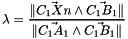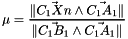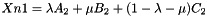Parameters
 Tn Triangle_3 Tn1 Triangle_3 Xn Point_3
Warning
Specific coupling procedure !
Returns
Point_3
 Triangle_3 tr ( const Triangle_3 & Tn, const Triangle_3 & Tn1, const Triangle_3 & T )

Barycentric transformation of Triangle T.

Calls function tr(Triangle_3, Triangle_3, Point_3) for each triangle vertex.

Parameters
 Tn Triangle_3 Tn1 Triangle_3 T Triangle_3
Warning
Specific coupling procedure !
Returns
Triangle_3
 Point_2 tr ( const Triangle_3 & Tn1, const Point_3 & Xn )

Transformation of a Point_3 into a Point_2.

Let Triangle Tn1(A,B,C), the barycentric transform of Point_3 Xn (belonging to Tn1) into a Point_2 is given by: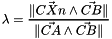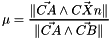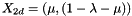Parameters
 Tn1 Triangle_3 Xn Point_3
Warning
Specific coupling procedure !
Returns
Point_2
 Triangle_2 tr ( const Triangle_3 & Tn1, const Triangle_3 & T )

Transformation of a Triangle_3 into a Triangle_2.

Calls function tr(Triangle_3, Point_3) for each triangle vertex.

Parameters
 Tn1 Triangle_3 T Triangle_3
Warning
Specific coupling procedure !
Returns
Triangle_2
 Point_3 tr ( const Triangle_3 & Tn1, const Point_2 & Xn )

Barycentric transformation of a Point_2 into a Point_3.

Let triangle Tn1(A,B,C), the transform of Point_2 Xn(X,Y) into a point_3 (belonging to Tn1) is given by: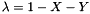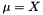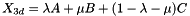Parameters
 Tn1 Triangle_3 Xn Point_2
Warning
Specific coupling procedure !
Returns
Point_3
 Triangle_3 tr ( const Triangle_3 & Tn1, const Triangle_2 & T )

Barycentric transformation of a Triangle_2 into a Triangle_3.

Calls function tr(Triangle_3, Point_2) for each triangle vertex.

Parameters
 Tn1 Triangle_3 T Triangle_2
Warning
Specific coupling procedure !
Returns
Triangle_3
 double volume_prisme ( const Triangle_3 & T1, const Triangle_3 & T2 )

Computation of the signed volume of a prism.

The signed volume of the prism with bases the triangles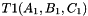and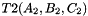is given by: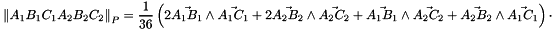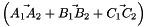Parameters
 T1 Triangle_3 basis of the prism T2 Triangle_3 basis of prism
Warning
Specific coupling procedure !
Returns
double
 double volume_tetra ( const Tetrahedron & Tet )

Computation of the signed colume of a tetrahedron.

The signed volume of a tetrahedronis given by: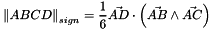Parameters
 Tet Tetrahedron
Warning
Specific coupling procedure !
Returns
double